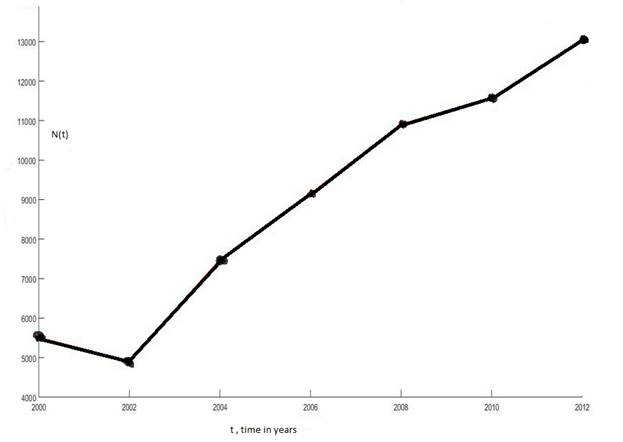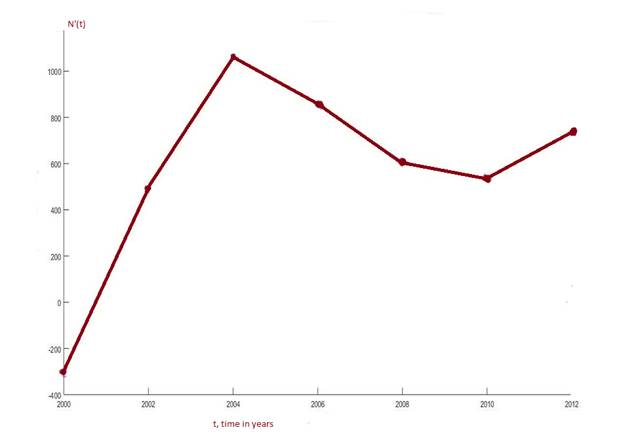Chapter 2.2, Problem 34E

Chapter
Section
Textbook Problem
52 views

# The table gives the number N ( t ) , measured in thousands, of minimally invasive cosmetic surgery procedures performed in the United States for various years t. t N(t) (thousands) 2000 5, 500 2002 4, 897 2004 7, 470 2006 9, 138 2008 10, 89 2010 11, 561 2012 13, 035 Source: American Society of Plastic Surgeons (a) What is the meaning of N ′ ( t ) ? What are its units? (b) Construct a table of estimated values for N ′ ( t ) . (c) Graph N and N ′ . (d) How would it be possible to get more accurate values for N ′ ( t ) ?

(a)

To determine

To write:

the meaning of  N'(t).

The meaning of N't is the rate of change of cosmetic surgery procedures with respect to time. And its unit is number of surgery procedures (in thousands) per year.

(b)

To find:

Table of N't

 t N(t)  (in thousands) N't 2000 5500 -301.5 2002 4897 1286.5 2004 7470 834 2006 9138 879.5 2008 10897 332 2010 11561 737 2012 13035

Explanation:

Formula:

N't   = Nt+h   -Nth, taking h = 2

Given: The table of Nt is given

Calculation:

We can find the values of N't by using the rate of change formula N't= Nt+h   -Nth

Where t = time is years and h = change in years

N'2000= N2002-N(2000)2=-301.5

N'2002= N2004-N(2002)2=1286.5

N'2004= N2006-N(2004)2= 834

N'2006= N2008-N(2006)2=879.5

N'2008=  N2010  - N(2008)2  = 332

N'2010= N2012-N(2010)2= 737

(c)

To graph:

N(t) and N’(t) versus tGraph of N'(t)(d) To explain:

how will you be able to find accurate values of N(t).

In part (b) we have calculated average rate of change in Nt.  To get more accurate value of N'(t) using the given data, we may compute two different values of rate of change for each year by using the  two different values for h namely h = 2 and h = -2, and take average of both values. Notice that this cannot be done at the end points.Thus N't   = 1/2(Nt+2   -Nt2 +Nt-2   -Nt-2 )

A more correct approach would to be sub divide the time to as small units as possible. But we do not have that data.

Explanation

Formula:

N't   = Nt+h   -Nth, taking h = 2

Given: The table of Nt is given

Calculation:

We can find the values of N't by using the rate of change formula N't= Nt+h   -Nth

Where t = time is years and h = change in years

N'2000= N2002-N(2000)2=-301.5

N'2002= N2004-N(2002)2=1286.5

N'2004= N2006-N(2004)2= 834

N'2006= N2008-N(2006)2=879

### Still sussing out bartleby?

Check out a sample textbook solution.

See a sample solution

#### The Solution to Your Study Problems

Bartleby provides explanations to thousands of textbook problems written by our experts, many with advanced degrees!

Get Started

#### Convert the expressions in Exercises 6584 to power form. 35x5x8+72x3

Finite Mathematics and Applied Calculus (MindTap Course List)

#### Evaluate the definite integrals in Problems 1-32. 20.

Mathematical Applications for the Management, Life, and Social Sciences

#### In Exercises 23-30, use logarithms to solve the equation for t. 5e2t=6

Finite Mathematics for the Managerial, Life, and Social Sciences

#### What is a ceiling effect, and how can it be a problem?

Research Methods for the Behavioral Sciences (MindTap Course List)